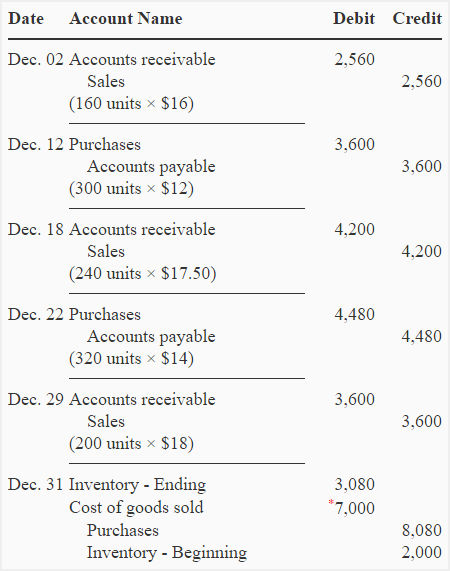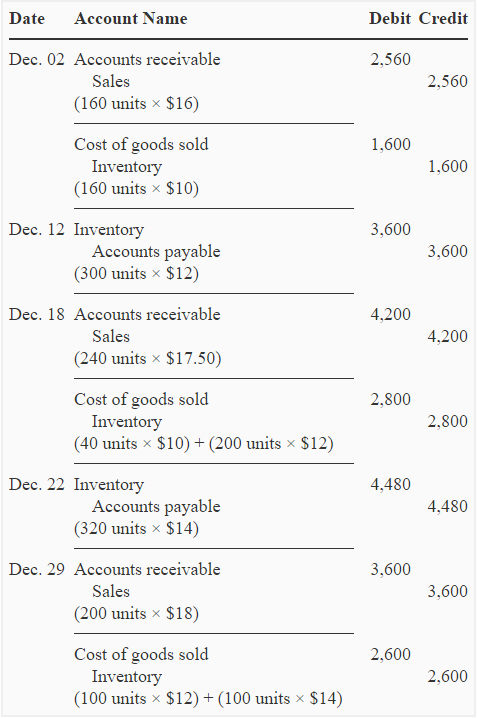# Exercise-7 (Periodic inventory system vs perpetual inventory system)

The Pharma company is a single product company. The company presents the following information regarding its activities during the month of December 2013.

• Dec. 01: Beginning inventory; 200 units @ \$10 each.
• Dec. 02: Sold 160 units @ \$16 each.
• Dec. 12: Purchased 300 units @ \$12 each.
• Dec. 18: Sold 240 units @ \$17.50 each.
• Dec. 22: Purchased 320 units @ \$14 each.
• Dec. 29: Sold 200 units @  \$18 each.

At the end of December, there were 220 units on hand according to a physical count of inventory.

Pharma company uses a first-in, first-out (FIFO) cost flow assumption. All purchases and sales are made on account.

Required:

1. Prepare journal entries and compute gross profit assuming the company uses a periodic inventory system.
2. Prepare journal entries and compute gross profit assuming the company uses a perpetual inventory system.

## Solution:

### (1). If periodic inventory method is used:

(a). Journal entries:*Balancing figure: (2,000 + 8,080) – 3,080 = 7,000

(b). Computation of gross profit:

Gross profit = Sales – Cost of goods sold

= \$10,360* – \$7,000**

= \$3,360

*(2,560 + 4,200 + 3,600)

**See last journal entry in part ‘a’

### (2). If perpetual inventory method is used:

(a). Journal entries:(b). Computation of gross profit:

Gross profit = Sales – Cost of goods sold

= \$10,360* – \$7,000**

= \$3,360

*(2,560 + 4,200 + 3,600)

**(1,600 + 2,800 + 2,600)

Notice that cost of goods sold and gross profit of the Pharma company are same under both periodic and perpetual inventory methods. It is because of the use of FIFO cost flow assumption.

A D V E R T I S E M E N T
4 Comments on Exercise-7 (Periodic inventory system vs perpetual inventory system)
1.Thank you limitless, it is strongly useful lesson.

2.Mupenzi

How useful and helpful the lessons are for accounting beginners ! Cheers!!!!

3.4.